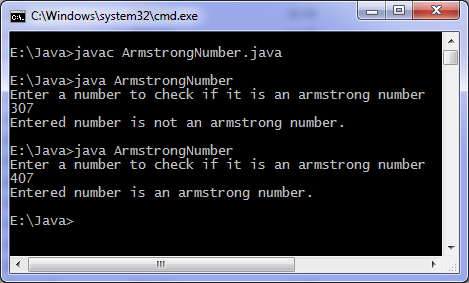# To check armstrong number

Levels of difficulty: / perform operation:

This java program checks if a number is armstrong or not.

## Java Example

```import java.util.*;

class ArmstrongNumber
{
public static void main(String args[])
{
int n, sum = 0, temp, r;

Scanner in = new Scanner(System.in);
System.out.println("Enter a number to check if it is an armstrong number");
n = in.nextInt();

temp = n;

while( temp != 0 )
{
r = temp%10;
sum = sum + r*r*r;
temp = temp/10;
}

if ( n == sum )
System.out.println("Entered number is an armstrong number.");
else
System.out.println("Entered number is not an armstrong number.");
}
}
```

### OutputUsing one more loop in the above code you can generate armstrong numbers from 1 to n(say) or between two integers (a to b).